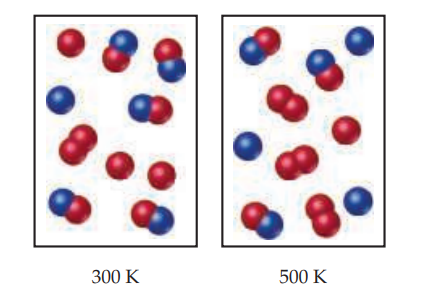×
Get Full Access to Chemistry: The Central Science - 14 Edition - Chapter 15 - Problem 15.11
Get Full Access to Chemistry: The Central Science - 14 Edition - Chapter 15 - Problem 15.11

×

# ?The following diagrams represent equilibrium mixtures for the reaction $$\mathrm{A}_{2}+\mathrm{B} \rightleftharpoons \mathrm{A}+\mathrm{AB}$$ at 300ISBN: 9780134414232 1274

## Solution for problem 15.11 Chapter 15

Chemistry: The Central Science | 14th Edition

• Textbook Solutions
• 2901 Step-by-step solutions solved by professors and subject experts
• Get 24/7 help from StudySoup virtual teaching assistantsChemistry: The Central Science | 14th Edition

4 5 1 299 Reviews
12
4
Problem 15.11

The following diagrams represent equilibrium mixtures for the reaction $$\mathrm{A}_{2}+\mathrm{B} \rightleftharpoons \mathrm{A}+\mathrm{AB}$$ at 300 K and 500 K. The A atoms are red, and the B atoms are blue. Is the reaction exothermic or endothermic? [Section 15.7]Text Transcription:

A_2 + B \rightleftharpoons A + AB

Step-by-Step Solution:

Step 1 of 5) In recent years fracking, short for hydraulic fracturing, has become widely used to greatly increase the availability of petroleum reserves. In fracking, a large volume of water, typically two million gallons or more, mixed with various additives, is injected at high pressure into wellbores extended horizontally into rock formations (Figure 18.23). The water is laden with sand, ceramic materials, and other additives, including gels, foams, and compressed gases, that serve to increase the yield in the process. The high-pressure fluid finds its way into tiny faults in geological formations, releasing petroleum and natural gas. Fracking has greatly increased petroleum reserves, particularly of natural gas, in many parts of the world. Unfortunately, the potential for environmental damage from fracking is significant. The large volume of fracking fluid required to create a well must be returned to the surface. Without purification the fluid is rendered unfit for other uses, and becomes a large-scale environmental problem. Often the waste water is allowed to sit in open waste water pits. The 2005 Energy Policy act and other federal legislation exempts hydraulic fracturing operations from certain provisions of the Safe Drinking Water act and other regulations.

Step 2 of 2

## Discover and learn what students are asking

Calculus: Early Transcendental Functions : Linear Models and Rates of Change
?In Exercises 5-10, plot the pair of points and find the slope of the line passing through them. (4,6), (4,1)

Calculus: Early Transcendental Functions : Inverse Trigonometric Functions: Integration
?In Exercises 1-20, find the indefinite integral. $$\int \frac{1}{\sqrt{1-(x+1)^{2}}} d x$$

Chemistry: The Central Science : Molecular Geometry and Bonding Theories
?How does a trigonal pyramid differ from a tetrahedron so far as molecular geometry is concerned?

Statistics: Informed Decisions Using Data : Tests for Independence and the Homogeneity of Proportions
?The table in the next column contains observed values and expected values in parentheses for two categorical variables, X and Y, where variable X has

Unlock Textbook Solution

?The following diagrams represent equilibrium mixtures for the reaction $$\mathrm{A}_{2}+\mathrm{B} \rightleftharpoons \mathrm{A}+\mathrm{AB}$$ at 300